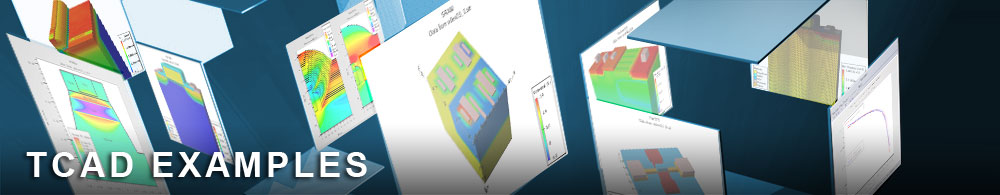Noise from a resistor

noiseex01.in : Noise from a resistor

Requires: S-Pisces/Noise
Minimum Versions: Atlas 5.28.1.R

This example shows a Noise simulation in Atlas.

A 10k n-type silicon resistor is modeled, and a DC_IV sweep is performed to confirm the resistance.

The noise generated by a device is modeled by taking an ideal (noiseless) device and adding a random voltage source to each port. For a one port device we have a single noise voltage source. The noise behavior is described by the statistical nature of these voltage sources. The mean value of the voltage from the source is zero and so the noise is described by the autocorrelation of the voltage source <v^2>.

Noise is an extension of the usual ac analysis of a device: a noise simulation can be performed on any device on which ac analysis can be performed. On the log command we have to define which contact is the principle for the inport and we have to add the keyword noise.v to tell Atlas to calculate the statistical properties of the noise voltage sources.

On the solve command we use the noise.ss keyword to tell Atlas to calculate the noise over the defined frequency range. N.b. the direct method must be used when calculating the noise.

The Nyquist theorem gives the expected noise generated by a resistor as <v^2>=4kTR, where k is Boltzmann's constant, T is the temperature of the resistor, and R is the resistance. For a 10k resistor at 300K this gives <v^2>=1.657e-16 V^2/Hz which agrees well with the result of the simulation.

To load and run this example, select the Load button in DeckBuild > Examples. This will copy the input file and any support files to your current working directory. Select the Run button in DeckBuild to execute the example.